### Home > CALC > Chapter 3 > Lesson 3.4.2 > Problem3-167

3-167.
1. Define f(x) and g(x) so that h(x) = f(g(x)), for the following functions given that f(x) ≠ x and g(x) ≠ x. Homework Help ✎

1. h(x) = cos(3x − 11)

2.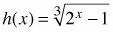3. h(x) = 35−2x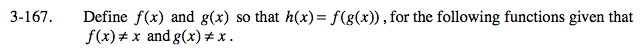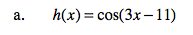g(x) = 3x − 11, the inner function.
f(x) = cosx, the outer function.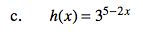Refer to hints above.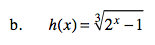2x − 1 is the inner function.
_____________ is the outer function.